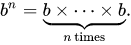# Exponents free online calculator

Online exponents calculator with negative numbers support and steps.

* Use e for scientific notation. E.g: 5e3, 4e-8, 1.45e12

** To find the exponent from the base and the exponentation result, use:

Logarithm calculator »

## Exponents laws and rules

The exponent formula is:

a n = a×a×...×a

n times

The base a is raised to the power of n, is equal to n times multiplication of a.

For example:

25 = 2×2×2×2×2 = 32

##### Multiplying exponents

an am = an+m

Example: 23 ⋅ 24 = 2(3+4) = 27 = 128

an bn = (a b) n

Example: 32 ⋅ 42 = (3⋅4)2 = 122 = 144

##### Dividing exponents

an / am = an-m

Example: 25 / 23 = 2(5-3) = 22 = 4

an / bn = (a / b) n

Example: 82 / 22 = (8/2)2 = 42 = 16

##### Power of exponent

(an)m = anm

Example: (23)4 = 2(3 ⋅ 4) = 212 = 4096

m√(an) = an/m

Example: 2√(26) = 2(6 / 2) = 23 = 8

##### Negative exponent

a -n = 1 / a n

Example: 2-3 = 1 / 23 = 1 / 8 = 0.125

##### Zero exponent

a 0 = 1

Example: 40 = 1

See: exponents rules

## Exponentiation definition

Exponentiation is a mathematical operation, written as bn, involving two numbers, the base b and the exponent or power n, and pronounced as "b raised to the power of n". When n is a positive integer, exponentiation corresponds to repeated multiplication of the base: that is, bn is the product of multiplying n bases:The exponent is usually shown as a superscript to the right of the base. In that case, bn is called "b raised to the nth power", "b raised to the power of n", "the nth power of b", "b to the nth power", or most briefly as "b to the nth".

One has b1 = b, and, for any positive integers m and n, one has bn ⋅ bm = bn+m. To extend this property to non-positive integer exponents, b0 is defined to be 1, and b-n (with n a positive integer and b not zero) is defined as 1/bn . In particular, b-1 is equal to 1/b, the reciprocal of b.

The definition of exponentiation can be extended to allow any real or complex exponent. Exponentiation by integer exponents can also be defined for a wide variety of algebraic structures, including matrices.

Exponentiation is used extensively in many fields, including economics, biology, chemistry, physics, and computer science, with applications such as compound interest, population growth, chemical reaction kinetics, wave behavior, and public-key cryptography.

Logarithm calculator »

Currently, we have around 1975 calculators, conversion tables and usefull online tools and software features for students, teaching and teachers, designers and simply for everyone.